See A.2 here and here for the source of this data.

It consists of two datasets, A and B, of 5000 records each, with each record in dataset A having a corresponding record in dataset B. The aim will be to capture as many of those 5000 true links as possible, with minimal false linkages.

It is worth noting that we should not necessarily expect to capture all links. There are some links that although we know they do correspond to the same person, the data is so mismatched between them that we would not reasonably expect a model to link them, and indeed should a model do so may indicate that we have overengineered things using our knowledge of true links, which will not be a helpful reference in situations where we attempt to link unlabelled data, as will usually be the case.

### Exploring data and defining model¶

Firstly let's read in the data and have a little look at it

``````import pandas as pd
import altair as alt
from IPython.display import IFrame
alt.renderers.enable('mimetype')

fn,
delimiter=", ",
dtype={"date_of_birth": str},
engine="python"
)
df["cluster"] = df["rec_id"].apply(lambda x: "-".join(x.split('-')[:2]))
return df
dfs = [
for dataset in ["a", "b"]
]

``````
rec_id given_name surname street_number address_1 address_2 suburb postcode state date_of_birth soc_sec_id cluster
0 rec-1070-org michaela neumann 8.0 stanley street miami winston hills 4223 nsw 19151111 5304218 rec-1070
1 rec-1016-org courtney painter 12.0 pinkerton circuit bega flats richlands 4560 vic 19161214 4066625 rec-1016
rec_id given_name surname street_number address_1 address_2 suburb postcode state date_of_birth soc_sec_id cluster
0 rec-561-dup-0 elton NaN 3.0 light setreet pinehill windermere 3212 vic 19651013 1551941 rec-561
1 rec-2642-dup-0 mitchell maxon 47.0 edkins street lochaoair north ryde 3355 nsw 19390212 8859999 rec-2642

Next, to better understand which variables will prove useful in linking, we have a look at how populated each column is, as well as the distribution of unique values within each

``````from splink.duckdb.duckdb_linker import DuckDBLinker

basic_settings = {
"unique_id_column_name": "rec_id",
# NB as we are linking one-one, we know the probability that a random pair will be a match
# hence we could set:
# "probability_two_random_records_match": 1/5000,
# however we will not specify this here, as we will use this as a check that
# our estimation procedure returns something sensible
}

``````
``````linker.missingness_chart()
``````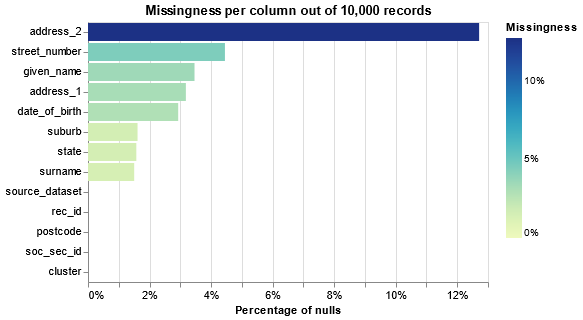``````cols_to_profile = list(dfs.columns)
cols_to_profile = [col for col in cols_to_profile if col not in ("rec_id", "cluster")]
``````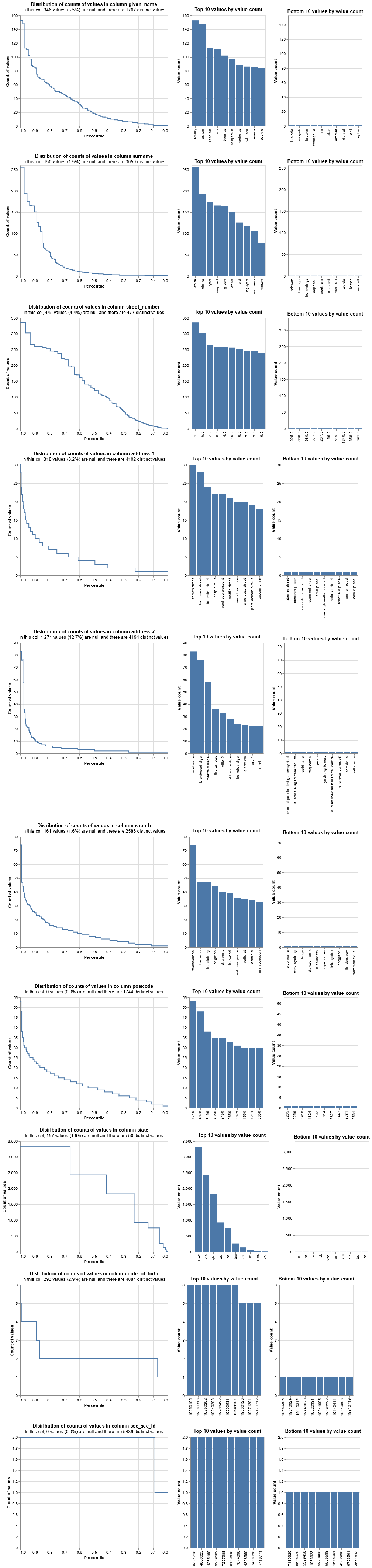Next let's come up with some candidate blocking rules, which define which record comparisons are generated, and have a look at how many comparisons each will generate.

For blocking rules that we use in prediction, our aim is to have the union of all rules cover all true matches, whilst avoiding generating so many comparisons that it becomes computationally intractable - i.e. each true match should have at least one of the following conditions holding.

``````blocking_rules = [
"l.given_name = r.given_name AND l.surname = r.surname",
"l.date_of_birth = r.date_of_birth",
"l.soc_sec_id = r.soc_sec_id",
"l.postcode = r.postcode",
]
``````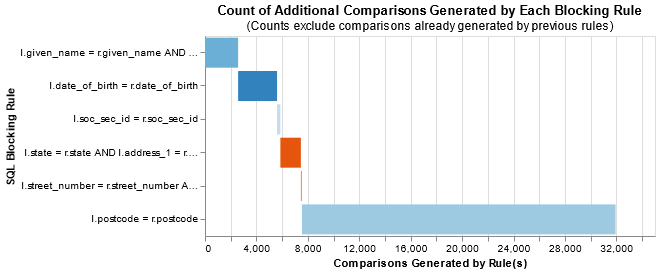The broadest rule, having a matching postcode, unsurpisingly gives the largest number of comparisons. For this small dataset we still have a very manageable number, but if it was larger we might have needed to include a further `AND` condition with it to break the number of comparisons further.

Now we get the full settings by including the blocking rules, as well as deciding the actual comparisons we will be including in our model.

We will define two models, each with a separate linker with different settings, so that we can compare performance. One will be a very basic model, whilst the other will include a lot more detail.

``````import splink.duckdb.duckdb_comparison_level_library as cll

# the simple model only considers a few columns, and only two comparison levels for each
simple_model_settings = {
**basic_settings,
"blocking_rules_to_generate_predictions": blocking_rules,
"comparisons": [
],
"retain_intermediate_calculation_columns": True,
}
# the detailed model considers more columns, using the information we saw in the exploratory phase
# we also include further comparison levels to account for typos and other differences
detailed_model_settings = {
**basic_settings,
"blocking_rules_to_generate_predictions": blocking_rules,
"comparisons": [
{
"output_column_name": "Given name",
"comparison_levels": [
cll.null_level("given_name"),
cll.columns_reversed_level("given_name", "surname"),
cll.distance_function_level("given_name", "jaro_winkler_similarity", 0.9),
cll.distance_function_level("given_name", "jaro_winkler_similarity", 0.7),
cll.else_level(),
],
},
{
"output_column_name": "Surname",
"comparison_levels": [
cll.null_level("surname"),
cll.distance_function_level("surname", "jaro_winkler_similarity", 0.9),
cll.distance_function_level("surname", "jaro_winkler_similarity", 0.7),
cll.else_level(),
],
},
cl.levenshtein_at_thresholds(
),
cl.levenshtein_at_thresholds("soc_sec_id", [1, 2]),
# we don't consider further location columns as they will be strongly correlated with postcode
],
"retain_intermediate_calculation_columns": True,
}

``````

### Estimating model parameters¶

We need to furnish our models with parameter estimates so that we can generate results. We will focus on the detailed model, generating the values for the simple model at the end

We can instead estimate the probability two random records match, and compare with the known value of 1/5000 = 0.0002, to see how well our estimation procedure works.

To do this we come up with some deterministic rules - the aim here is that we generate very few false positives (i.e. we expect that the majority of records with at least one of these conditions holding are true matches), whilst also capturing the majority of matches - our guess here is that these two rules should capture 80% of all matches.

``````deterministic_rules = [
"l.soc_sec_id = r.soc_sec_id",
"l.given_name = r.given_name and l.surname = r.surname and l.date_of_birth = r.date_of_birth",
]

``````
``````Probability two random records match is estimated to be  0.000238.
This means that amongst all possible pairwise record comparisons, one in 4,195.51 are expected to match.  With 25,000,000 total possible comparisons, we expect a total of around 5,958.75 matching pairs
```
```

Even playing around with changing these deterministic rules, or the nominal recall leaves us with an answer which is pretty close to our known value

Next we estimate `u` and `m` values for each comparison, so that we can move to generating predictions

``````linker_detailed.estimate_u_using_random_sampling(target_rows=1e7)
``````
``````----- Estimating u probabilities using random sampling -----

Estimated u probabilities using random sampling

Your model is not yet fully trained. Missing estimates for:
- Given name (no m values are trained).
- Surname (no m values are trained).
- date_of_birth (no m values are trained).
- soc_sec_id (no m values are trained).
- street_number (no m values are trained).
- postcode (no m values are trained).
```
```

When training the `m` values using expectation maximisation, we need somre more blocking rules to reduce the total number of comparisons. For each rule, we want to ensure that we have neither proportionally too many matches, or too few.

We must run this multiple times using different rules so that we can obtain estimates for all comparisons - if we block on e.g. `date_of_birth`, then we cannot compute the `m` values for the `date_of_birth` comparison, as we have only looked at records where these match.

``````session_dob = linker_detailed.estimate_parameters_using_expectation_maximisation(
"l.date_of_birth = r.date_of_birth"
)
"l.postcode = r.postcode"
)
``````
``````
----- Starting EM training session -----

Estimating the m probabilities of the model by blocking on:
l.date_of_birth = r.date_of_birth

Parameter estimates will be made for the following comparison(s):
- Given name
- Surname
- soc_sec_id
- street_number
- postcode

Parameter estimates cannot be made for the following comparison(s) since they are used in the blocking rules:
- date_of_birth

Iteration 1: Largest change in params was 0.529 in probability_two_random_records_match
Iteration 2: Largest change in params was 0.00321 in probability_two_random_records_match
Iteration 3: Largest change in params was 2.92e-05 in the m_probability of soc_sec_id, level `All other comparisons`

EM converged after 3 iterations

Your model is not yet fully trained. Missing estimates for:
- date_of_birth (no m values are trained).

----- Starting EM training session -----

Estimating the m probabilities of the model by blocking on:
l.postcode = r.postcode

Parameter estimates will be made for the following comparison(s):
- Given name
- Surname
- date_of_birth
- soc_sec_id
- street_number

Parameter estimates cannot be made for the following comparison(s) since they are used in the blocking rules:
- postcode

Iteration 1: Largest change in params was 0.0296 in the m_probability of date_of_birth, level `All other comparisons`
Iteration 2: Largest change in params was 0.000291 in the m_probability of date_of_birth, level `All other comparisons`
Iteration 3: Largest change in params was 4.43e-06 in the m_probability of soc_sec_id, level `All other comparisons`

EM converged after 3 iterations

Your model is fully trained. All comparisons have at least one estimate for their m and u values
```
```

If we wish we can have a look at how our parameter estimates changes over these training sessions

``````# session_dob.m_u_values_interactive_history_chart()
``````

For variables that aren't used in the `m`-training blocking rules, we have two estimates --- one from each of the training sessions (see for example `street_number`). We can have a look at how the values compare between them, to ensure that we don't have drastically different values, which may be indicative of an issue.

``````linker_detailed.parameter_estimate_comparisons_chart()
``````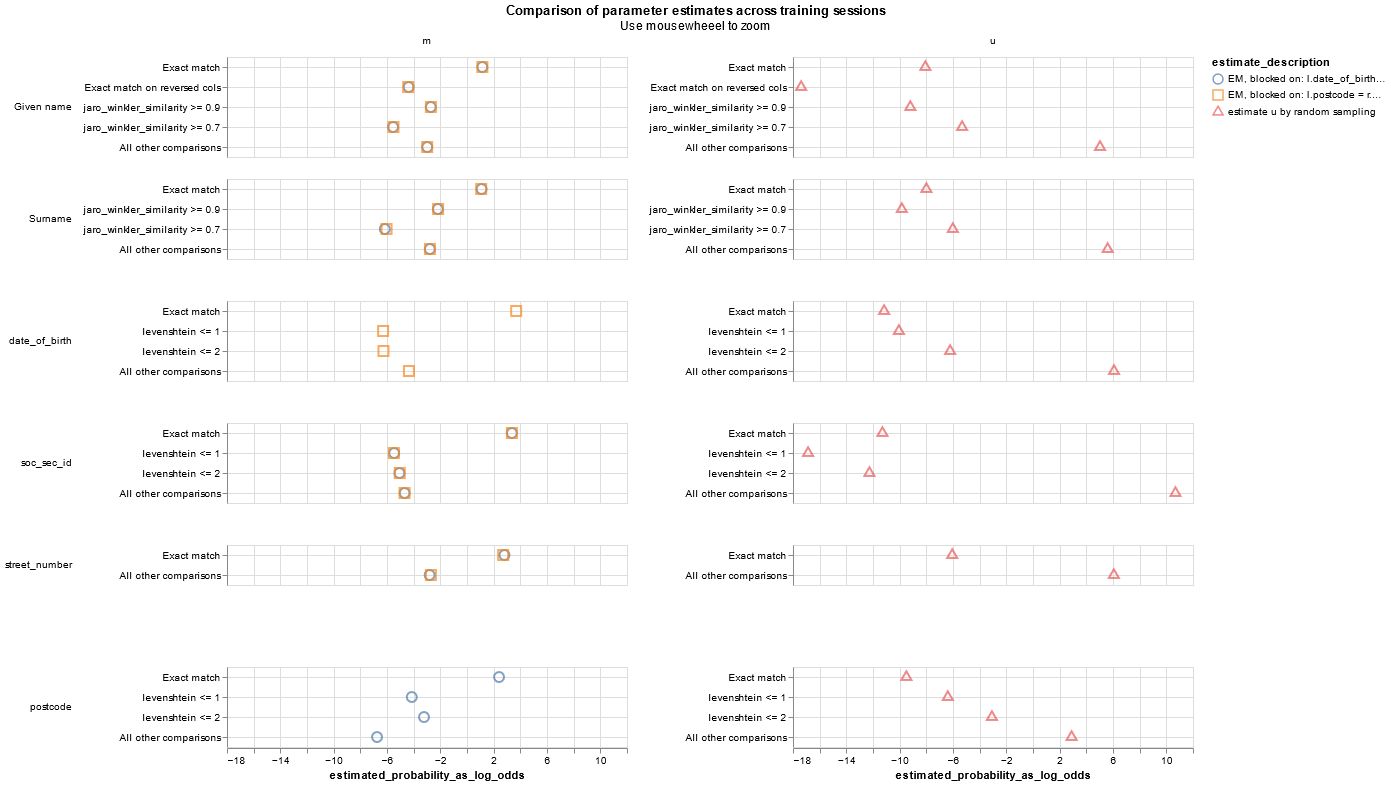We repeat our parameter estimations for the simple model in much the same fashion

``````linker_simple.estimate_probability_two_random_records_match(deterministic_rules, recall=0.8)
"l.given_name = r.given_name"
)
"l.street_number = r.street_number"
)
``````
``````Probability two random records match is estimated to be  0.000238.
This means that amongst all possible pairwise record comparisons, one in 4,195.51 are expected to match.  With 25,000,000 total possible comparisons, we expect a total of around 5,958.75 matching pairs
----- Estimating u probabilities using random sampling -----

Estimated u probabilities using random sampling

Your model is not yet fully trained. Missing estimates for:
- given_name (no m values are trained).
- surname (no m values are trained).
- street_number (no m values are trained).

----- Starting EM training session -----

Estimating the m probabilities of the model by blocking on:
l.given_name = r.given_name

Parameter estimates will be made for the following comparison(s):
- surname
- street_number

Parameter estimates cannot be made for the following comparison(s) since they are used in the blocking rules:
- given_name

Iteration 1: Largest change in params was -0.0987 in the m_probability of surname, level `Exact match`
Iteration 2: Largest change in params was -0.0471 in the m_probability of surname, level `Exact match`
Iteration 3: Largest change in params was 0.0331 in the m_probability of surname, level `All other comparisons`
Iteration 4: Largest change in params was 0.0219 in the m_probability of surname, level `All other comparisons`
Iteration 5: Largest change in params was -0.0138 in the m_probability of surname, level `Exact match`
Iteration 6: Largest change in params was -0.00855 in the m_probability of surname, level `Exact match`
Iteration 7: Largest change in params was -0.00527 in the m_probability of surname, level `Exact match`
Iteration 8: Largest change in params was 0.00325 in the m_probability of surname, level `All other comparisons`
Iteration 9: Largest change in params was -0.00202 in the m_probability of surname, level `Exact match`
Iteration 10: Largest change in params was 0.00126 in the m_probability of surname, level `All other comparisons`
Iteration 11: Largest change in params was -0.000793 in the m_probability of surname, level `Exact match`
Iteration 12: Largest change in params was -0.0005 in the m_probability of surname, level `Exact match`
Iteration 13: Largest change in params was -0.000316 in the m_probability of surname, level `Exact match`
Iteration 14: Largest change in params was -0.000201 in the m_probability of surname, level `Exact match`
Iteration 15: Largest change in params was 0.000128 in the m_probability of surname, level `All other comparisons`
Iteration 16: Largest change in params was -8.12e-05 in the m_probability of surname, level `Exact match`

EM converged after 16 iterations

Your model is not yet fully trained. Missing estimates for:
- given_name (no m values are trained).

----- Starting EM training session -----

Estimating the m probabilities of the model by blocking on:
l.street_number = r.street_number

Parameter estimates will be made for the following comparison(s):
- given_name
- surname

Parameter estimates cannot be made for the following comparison(s) since they are used in the blocking rules:
- street_number

Iteration 1: Largest change in params was 0.0656 in the m_probability of given_name, level `All other comparisons`
Iteration 2: Largest change in params was -0.0433 in the m_probability of given_name, level `Exact match`
Iteration 3: Largest change in params was 0.0278 in the m_probability of given_name, level `All other comparisons`
Iteration 4: Largest change in params was -0.0161 in the m_probability of given_name, level `Exact match`
Iteration 5: Largest change in params was 0.00883 in the m_probability of given_name, level `All other comparisons`
Iteration 6: Largest change in params was -0.00481 in the m_probability of given_name, level `Exact match`
Iteration 7: Largest change in params was 0.00265 in the m_probability of given_name, level `All other comparisons`
Iteration 8: Largest change in params was 0.00149 in the m_probability of given_name, level `All other comparisons`
Iteration 9: Largest change in params was -0.000869 in the m_probability of given_name, level `Exact match`
Iteration 10: Largest change in params was -0.000523 in the m_probability of given_name, level `Exact match`
Iteration 11: Largest change in params was -0.000325 in the m_probability of given_name, level `Exact match`
Iteration 12: Largest change in params was -0.000226 in the m_probability of surname, level `Exact match`
Iteration 13: Largest change in params was -0.000163 in the m_probability of surname, level `Exact match`
Iteration 14: Largest change in params was 0.000116 in the m_probability of surname, level `All other comparisons`
Iteration 15: Largest change in params was 8.19e-05 in the m_probability of surname, level `All other comparisons`

EM converged after 15 iterations

Your model is fully trained. All comparisons have at least one estimate for their m and u values
```
```
``````# import json
# we can have a look at the full settings if we wish, including the values of our estimated parameters:
# we can also get a handy summary of of the model in an easily readable format if we wish:
# (we suppress output here for brevity)
``````

We can now visualise some of the details of our models. We can look at the match weights, which tell us the relative importance for/against a match for each of our comparsion levels.

Comparing the two models will show the added benefit we get in the more detailed model --- what in the simple model is classed as 'all other comparisons' is instead broken down further, and we can see that the detail of how this is broken down in fact gives us quite a bit of useful information about the likelihood of a match.

``````linker_simple.match_weights_chart()
``````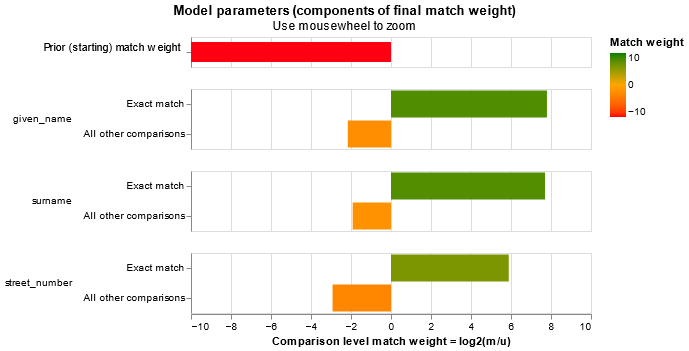``````linker_detailed.match_weights_chart()
``````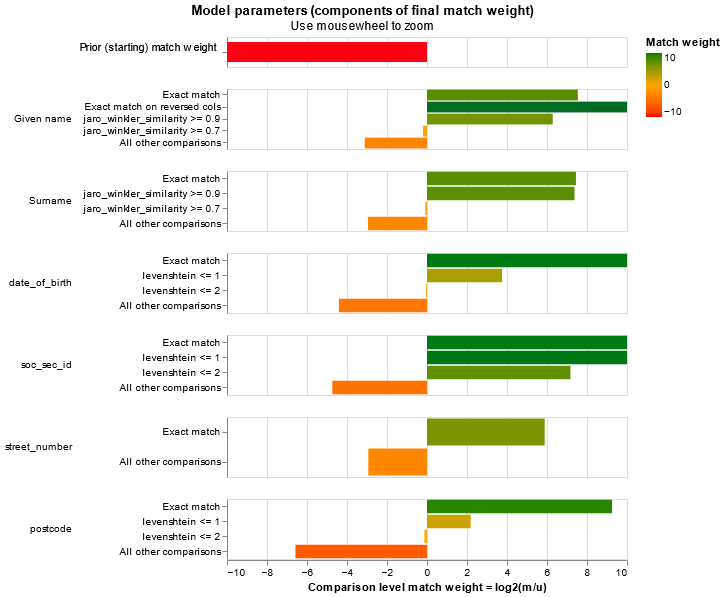As well as the match weights, which give us an idea of the overall effect of each comparison level, we can also look at the individual `u` and `m` parameter estimates, which tells us about the prevalence of coincidences and mistakes (for further details/explanation about this see this article). We might want to revise aspects of our model based on the information we ascertain here.

Note however that some of these values are very small, which is why the match weight chart is often more useful for getting a decent picture of things.

``````# linker_simple.m_u_parameters_chart()
``````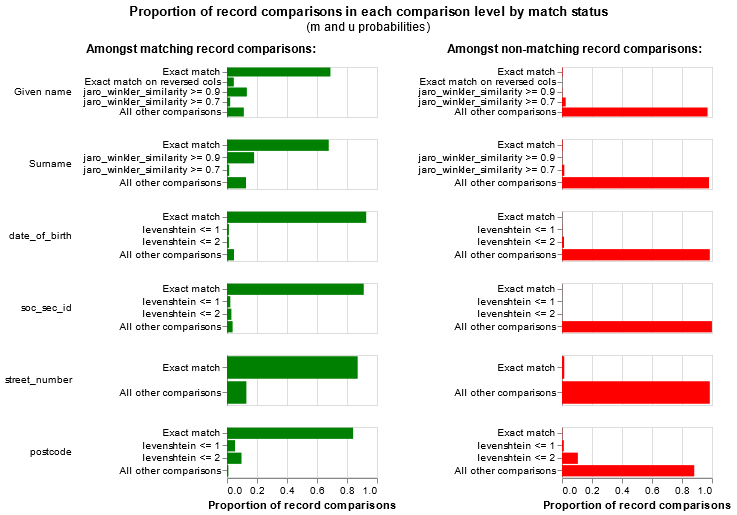It is also useful to have a look at unlinkable records - these are records which do not contain enough information to be linked at some match probability threshold. We can figure this out be seeing whether records are able to be matched with themselves.

This is of course relative to the information we have put into the model - we see that in our simple model, at a 99% match threshold nearly 10% of records are unlinkable, as we have not included enough information in the model for distinct records to be adequately distinguished; this is not an issue in our more detailed model.

``````linker_simple.unlinkables_chart()
``````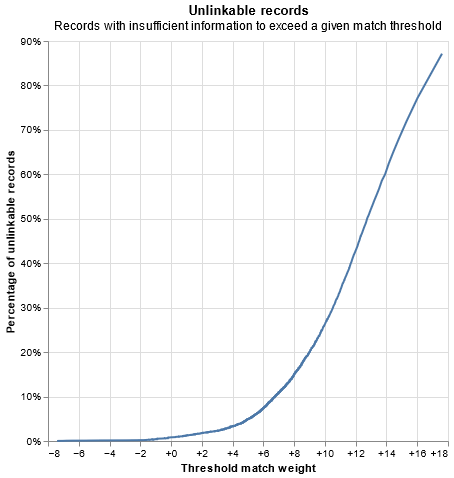``````linker_detailed.unlinkables_chart()
``````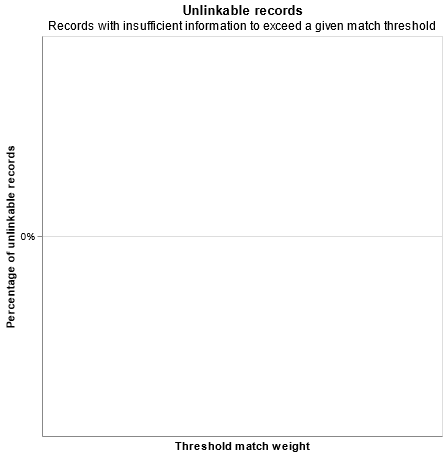Our simple model doesn't do terribly, but suffers if we want to have a high match probability --- to be 99% certain of matches we have ~10% of records that we will be unable to link.

Our detailed model, however, has enough nuance that we can at least self-link records.

### Predictions¶

Now that we have had a look into the details of the models, we will focus on only our more detailed model, which should be able to capture more of the genuine links in our data

``````predictions = linker_detailed.predict()
df_predictions = predictions.as_pandas_dataframe()
``````
match_weight match_probability source_dataset_l rec_id_l source_dataset_r rec_id_r given_name_l given_name_r surname_l surname_r ... bf_street_number bf_tf_adj_street_number postcode_l postcode_r gamma_postcode tf_postcode_l tf_postcode_r bf_postcode bf_tf_adj_postcode match_key
0 42.077751 1.0 _a rec-1016-org _b rec-1016-dup-0 courtney courtney painter painter ... 59.013018 0.647003 4560 4560 3 0.0030 0.0030 609.446252 0.459895 0
1 50.653290 1.0 _a rec-1288-org _b rec-1288-dup-0 vanessa vanessa parr parr ... 59.013018 70.523279 2135 2135 3 0.0015 0.0015 609.446252 0.919790 0
2 32.748771 1.0 _a rec-298-org _b rec-298-dup-0 blake blake howie howie ... 59.013018 0.418536 6017 6071 1 0.0005 0.0005 0.914304 1.000000 0
3 49.012108 1.0 _a rec-2404-org _b rec-2404-dup-0 blakeston blakeston broadby broadby ... 59.013018 4.029902 3083 3083 3 0.0011 0.0011 609.446252 1.254259 0
4 30.096869 1.0 _a rec-453-org _b rec-453-dup-0 edward edward denholm denholm ... 59.013018 0.548819 4221 4221 3 0.0017 0.0017 609.446252 0.811580 0

5 rows × 46 columns

We can see how our model performs at different probability thresholds, with a couple of options depending on the space we wish to view things

``````# linker_detailed.roc_chart_from_labels_column("cluster")
``````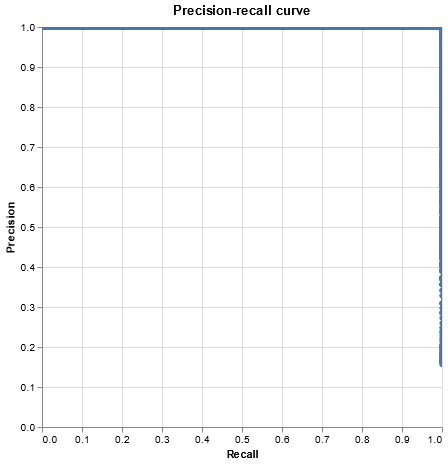and we can easily see how many individuals we identify and link by looking at clusters generated at some threshold match probability of interest - in this example 99%

``````clusters = linker_detailed.cluster_pairwise_predictions_at_threshold(predictions, threshold_match_probability=0.99)
df_clusters = clusters.as_pandas_dataframe().sort_values("cluster_id")
df_clusters.groupby("cluster_id").size().value_counts()
``````
``````Completed iteration 1, root rows count 0
```
```
``````2    4966
1      68
dtype: int64```
```

In this case, we happen to know what the true links are, so we can manually inspect the ones that are doing worst to see what our model is not capturing - i.e. where we have false negatives.

Similarly, we can look at the non-links which are performing the best, to see whether we have an issue with false positives.

Ordinarily we would not have this luxury, and so would need to dig a bit deeper for clues as to how to improve our model, such as manually inspecting records across threshold probabilities,

``````df_predictions["cluster_l"] = df_predictions["rec_id_l"].apply(lambda x: "-".join(x.split('-')[:2]))
df_predictions["cluster_r"] = df_predictions["rec_id_r"].apply(lambda x: "-".join(x.split('-')[:2]))
``````records_to_view = 3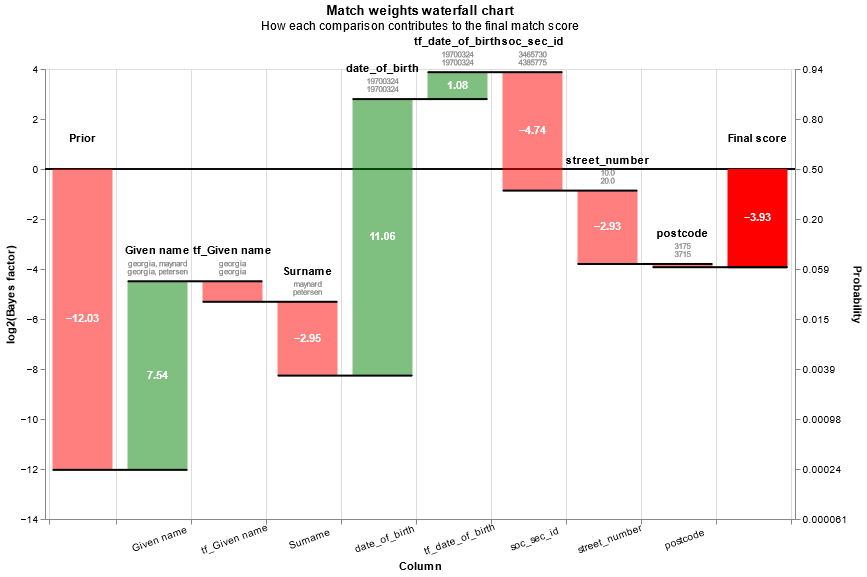``````df_non_links = df_predictions[df_predictions["cluster_l"] != df_predictions["cluster_r"]].sort_values("match_probability", ascending=False)